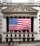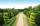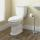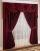# Fraction calculator

The calculator performs basic and advanced operations with fractions, expressions with fractions combined with integers, decimals, and mixed numbers. Also shows detailed step-by-step information about fraction calculation procedure. Solve problems with two, three or more fractions and numbers in one expression.

## Result:

### 1.5 - 11/5 = 3/10 = 0.3

Spelled result in words is three tenths.

### How do you solve fractions step by step?

1. Conversion a decimal number to a fraction: 1.5 = 1510 = 32

a) Write down the decimal 1.5 divided by 1: 1.5 = 1.51
b) Multiply both top and bottom by 10 for every number after the decimal point. (For example, if there are two numbers after the decimal point, then use 100, if there are three then use 1000, etc.)
1.51 = 1510
Note: 1510 is called a decimal fraction.

c) Simplify and reduce the fraction
1510 = 3 * 52 * 5 = 3 * 52 * 5 = 32
2. Conversion a mixed number 1 15 to a improper fraction: 1 1/5 = 1 15 = 1 · 5 + 15 = 5 + 15 = 65

To find new numerator:
a) Multiply the whole number 1 by the denominator 5. Whole number 1 equally 1 * 55 = 55
b) Add the answer from previous step 5 to the numerator 1. New numerator is 5 + 1 = 6
c) Write previous answer (new numerator 6) over the denominator 5.

One and one fifth is six fifths
3. Subtract: 1.5 - 65 = 32 - 65 = 3 · 52 · 5 - 6 · 25 · 2 = 1510 - 1210 = 15 - 1210 = 310
For adding, subtracting, and comparing fractions, it is suitable to adjust both fractions to a common (equal, identical) denominator. The common denominator you can calculate as the least common multiple of the both denominators - LCM(2, 5) = 10. In practice, it is enough to find the common denominator (not necessarily the lowest) by multiplying the denominators: 2 × 5 = 10. In the next intermediate step the fraction result cannot be further simplified by cancelling.
In words - three halfs minus six fifths = three tenths.

#### Rules for expressions with fractions:

Fractions - use the slash “/” between the numerator and denominator, i.e. for five-hundredths enter 5/100. If you are using mixed numbers be sure to leave a single space between the whole number and fraction part.
The slash separates the numerator (number above a fraction line) and denominator (number below).

Mixed numerals (mixed fractions or mixed numbers) write as non-zero integer separated by one space and fraction i.e., 1 2/3 (having the same sign). An example of a negative mixed fraction: -5 1/2.
Because slash is both signs for fraction line and division, we recommended use colon (:) as operator of division fractions i.e., 1/2 : 3.

Decimals (decimal numbers) enter with a decimal point . and they are automatically converted to fractions - i.e. 1.45.

Colon : and slash / is the symbol of division. Can be used to divide mixed numbers 1 2/3 : 4 3/8 or can be used for write complex fractions i.e. 1/2 : 1/3.
An asterisk * or × is the symbol for multiplication.
Plus + is addition, minus sign - is subtraction and ()[] is mathematical parentheses.
The exponentiation/power symbol is ^ - for example: (7/8-4/5)^2 = (7/8-4/5)2

#### Examples:

subtracting fractions: 2/3 - 1/2
multiplying fractions: 7/8 * 3/9
dividing Fractions: 1/2 : 3/4
exponentiation of fraction: 3/5^3
fractional exponents: 16 ^ 1/2
adding fractions and mixed numbers: 8/5 + 6 2/7
dividing integer and fraction: 5 ÷ 1/2
complex fractions: 5/8 : 2 2/3
decimal to fraction: 0.625
Fraction to Decimal: 1/4
Fraction to Percent: 1/8 %
comparing fractions: 1/4 2/3
multiplying a fraction by a whole number: 6 * 3/4
square root of a fraction: sqrt(1/16)
reducing or simplifying the fraction (simplification) - dividing the numerator and denominator of a fraction by the same non-zero number - equivalent fraction: 4/22
expression with brackets: 1/3 * (1/2 - 3 3/8)
compound fraction: 3/4 of 5/7
fractions multiple: 2/3 of 3/5
divide to find the quotient: 3/5 ÷ 2/3

Calculator follows well-known rules for order of operations. Most common mnemonics for remembering this order of operations are:
PEMDAS - Parentheses, Exponents, Multiplication, Division, Addition, Subtraction.
BEDMAS - Brackets, Exponents, Division, Multiplication, Addition, Subtraction
BODMAS - Brackets, Of or Order, Division, Multiplication, Addition, Subtraction.
GEMDAS - Grouping Symbols - brackets (){}, Exponents, Multiplication, Division, Addition, Subtraction.
Be careful, always do multiplication and division before addition and subtraction. Some operators (+ and -) and (* and /) has the same priority and then must evaluate from left to right.

## Fractions in word problems:

• SavingsEva borrowed 1/3 of her savings to her brother, 1/2 of savings spent in the store and 7 euros left. How much did she save?
• Two ribbonsThe total length of two ribbons is 13 meters. If one ribbon is 7 and 5/8 meters long, what is the length of the other ribbon.
• Wood 11Father has 12 1/5 meters long wood. Then I cut the wood into two pieces. One part is 7 3/5 meters long. Calculate the length of the other wood?
• RegroupingSubtract mixed number with regrouping: 11 17/20- 6 19/20
• Stock market 2For the week of July 22, the following day to day changes in the stock market was recorded a certain stock: -2 on Monday; +4 Tuesday; -8 Wednesday; + 2 1/2 Thursday; - 3 1/4 Friday. The stock began the week at 78 points. How many points did it finish with
• Bitoo and ReenaBitoo ate 3/5 part of an apple and the remaining part was eaten by his sister Reena. How much part of an apple did Renna eat? Who had the larger share? By how much?
• EricaErica bought 3 1/2 yards of fabric. If she uses 2/3 of the fabric, how much will she have left?
• Pounds3 pounds subtract 1/3 of a pound.
• Algebra problemThis is algebra. Let n represent an unknown number. 1. Eight more than the number n 2. Three times the number n 3. The product of the number n and eight 4. Three less than the number n 5. Three decreased by the number n
• GardenThe garden has a rectangular shape with lengths of 25 and 40 meters. It has been expanded so that each of its size increased by one fifth. How many square meters increased its acreage?
• PediatricianPediatrician this month of 20 working days takes 8 days holidays. What is the probability that on Monday will be at work?
• ToiletsA federal law requires that all residential toilets sold in the United States use no more than 1 3/5 gallons of water per flush. Prior to this legislation, conventional toilets used 3 2/5 gallons of water per flush. Find the amount of water saved in one y
• Interior designerTo make draperies an interior designer needs 11 1/4 yards of material for the den and 8 1/2 yards for the living room. If material comes only in 20 yard bolts, how much will be left over after completing both sets of draperies?